# A Deep Learning Approach for Native Language Identification (NLI)

#### Machine Learning (ML) Natural Language Processing (NLP)

By definition, Native-language identification (NLI) is the task of determining an author's native language based only on their writings or speeches in a second language. In this article, we will implement a model to identify native language of the author.

Introduction

PS: If you don't know what NLI is, I would recommend you to go to this link to understand the basics before you read the implementation.

NLI can be approached as a kind of text classification. We would be using BERT for this task.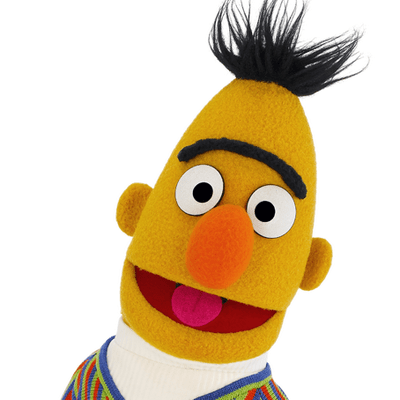BERT (Bidirectional Encoder Representations from Transformers) is a recent paper published by researchers at Google AI Language. BERT makes use of Transformer, an attention mechanism that learns contextual relations between words (or sub-words) in a text. In its vanilla form, Transformer includes two separate mechanisms — an encoder that reads the text input and a decoder that produces a prediction for the task. Since BERT’s goal is to generate a language model, only the encoder mechanism is necessary.

Here the task is to classify text i.e., predict author's native language based on the text given. So, classification can be done by adding a classifier or a SoftMax layer on top of BERT which gives a probability of all the classes and we take the max of it (the most likely class). This is because BERT takes text as an input and passes it through various encoders and gives us some finite length encoding of that text. That encoding represents that particular sentence.

Let us dive deeper to see how BERT works.

How BERT works

So, BERT is a model which was trained on BooksCorpus (800M words) and English Wikipedia (2.5B words) by google.

BERT used two training strategies (or how it is trained), one is Masked LM and the other is Next setence prediction.

Before feeding word sequences into BERT, 15% of the words in each sentence are replaced with a masked. This means that it is converted to a token which is called "masked token". Then the job of BERT is to predict that hidden or masked word in the sentence by looking at the words (non-masked words) around that masked word.The model then attempts to predict the original value of the masked words, based on the context provided by the other, non-masked, words in the sequence.

In simple terms, 15% of the words will be masked. It will be passed through BERT which will encode (it is a coded form which is different from input) it at the output. Then, that encoded form represents our sentence. Now, we can pass that encoded form to a classifier which is predict the most likely word that should be present in the masked location (or what "masked token" should to replaced with). We will continue this process again and again till BERT learns to output the correct word that we masked.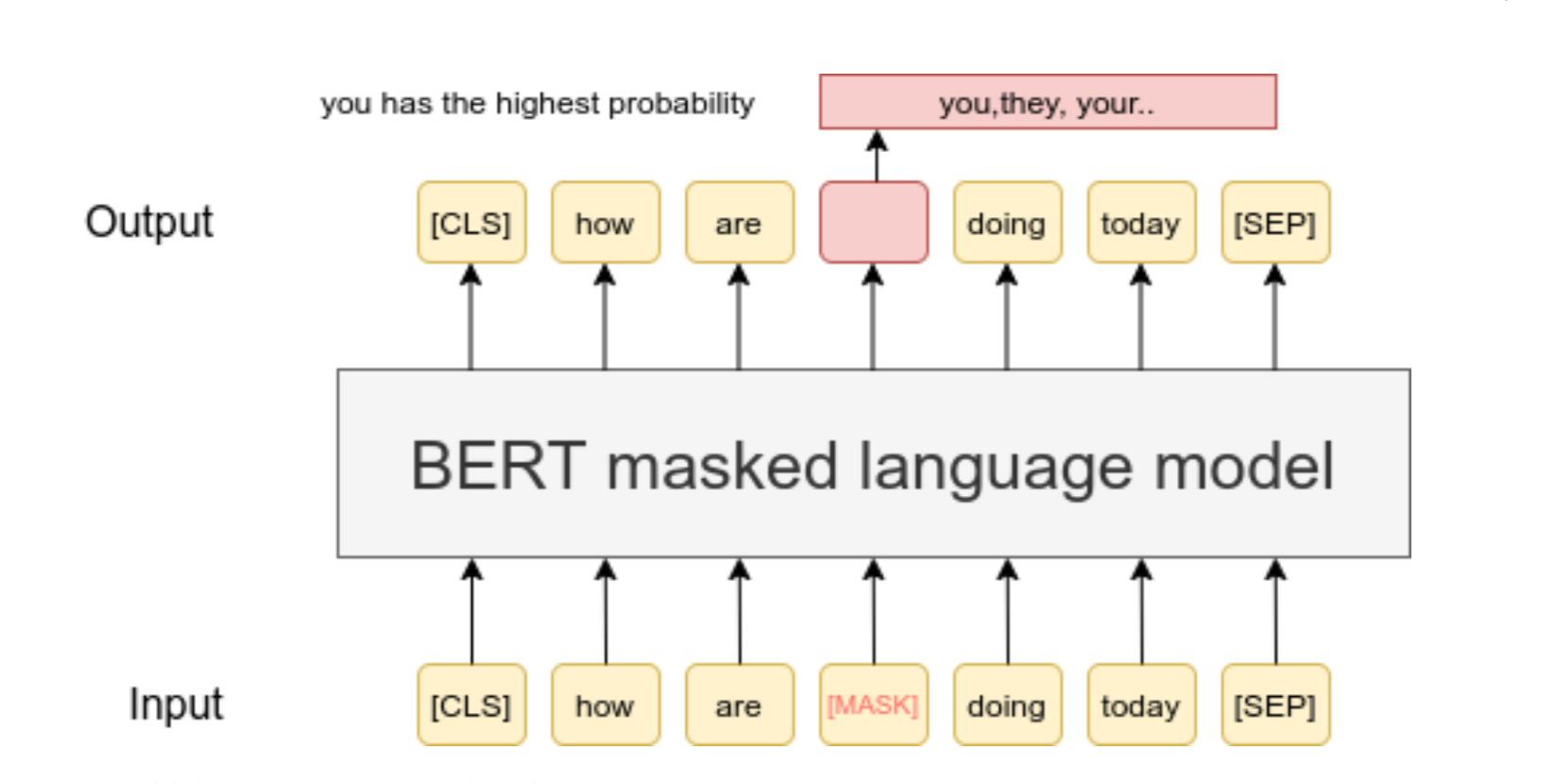In technical terms:

1. Adding a classification layer on top of the BERT output (such as a SVM).
2. Multiplying the output of BERT by a matrix, and then transforming them into our vocabulary size.
3. Calculating the probability of each word in the vocabulary with softmax and picking up the word that has the highest probability.

Next sentence prediction

Here, BERT is trained by Next sentence prediction strategy. In this training process, BERT receives pairs of sentences as input and learns to predict if the second sentence in the pair of the first sentence (which means that the second sentence occurs just after the first sentence in our training corpus). During training, 50% of the inputs are pairs in which the second sentence is the the pair of first sentence, while in the other 50%, it is just a random sentence from the corpus which is chosen as a second sentence. That means the other 50% doesn't forms a pair.

So, the job of BERT is to just classify the pair of sentence into two classes, whether it is a pair or not a pair. It is done by appending the binary classifier on top of BERT.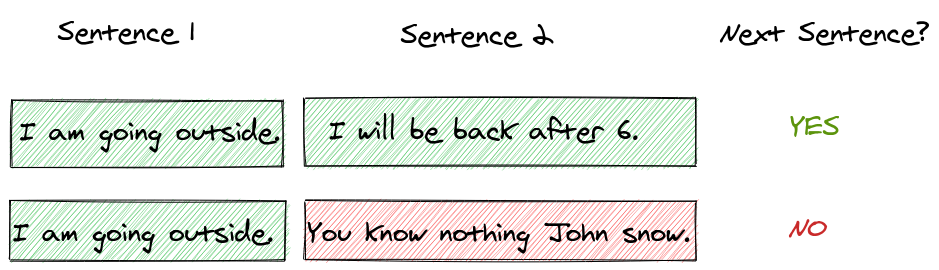So, a question arises is that what is the need to train BERT or any model in this fashion. It is never our goal to predict the masked words!

So, the answer is that, this process teaches BERT how English or any language is written. It learns the rules related to grammar, which words should be used where and so on. So, after training BERT becomes an expert in English language. It now know all the rules that are there in English language. This knowledge could be easily transferred to other NLP tasks and BERT has proven that by giving highest accuracies on benchmark tasks and thus producing state-of-the-art results.

Architecture of BERT

BERT is basically is layers of encoder stacked on top of each other.There are two version of BERT: BERTBASE has 12 layers in the Encoder stack while BERTLARGE has 24 layers in the Encoder stack.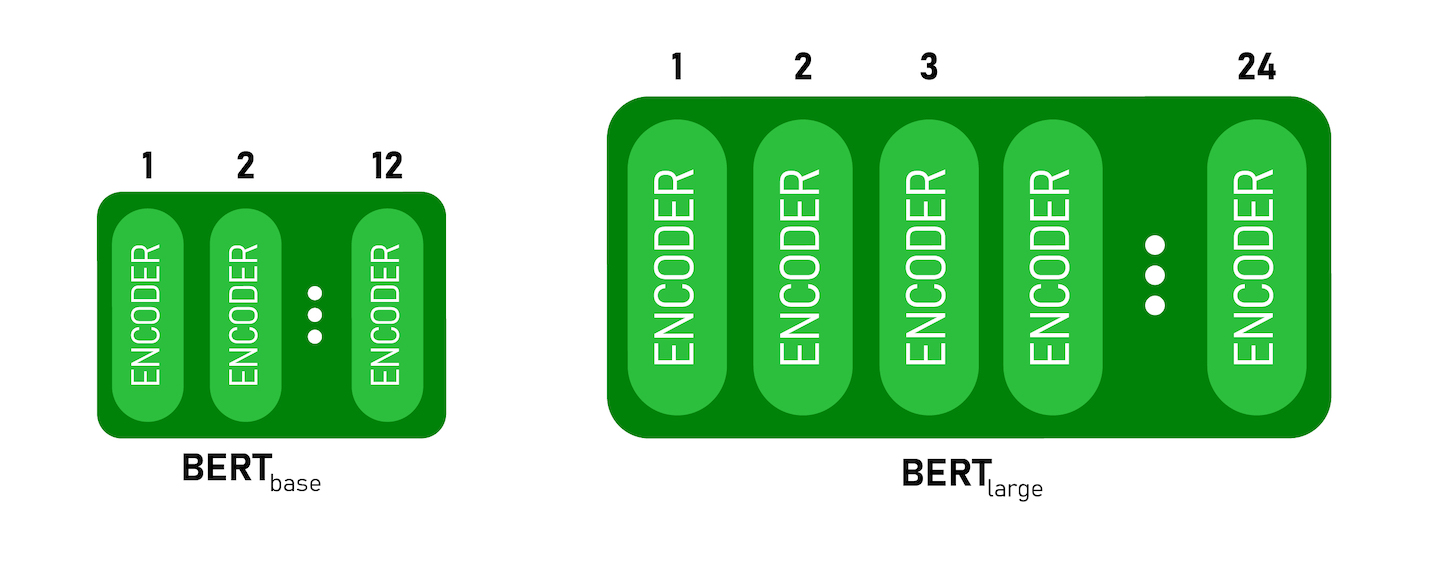Now, let us walk from input to output of BERT. BERT first divided the sentence into list of tokens of a particular size. So, if a sentence is short it is padded with a predefined token or if it is large, then some of them are removed. Diagram shows that after the sentence "I like to draw", padding tokens are added to it just to make the input length of a pre-defined size. Notice that there are two other tokens CLS and SEP besides PAD tokens. CLS informs the BERT that this location is the start of the sentence and SEP shows the end of the sentence.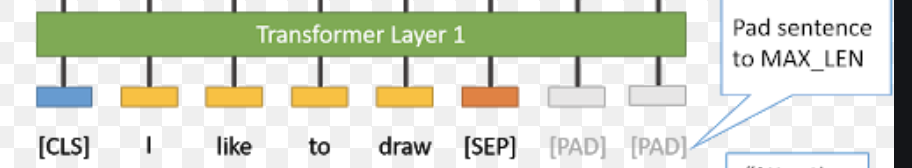Then each token has a particular vector. This vector is extracted from the BERT predefined vocab which consists of around 30K words. But what if some words are not there in the vocab. Then BERT breaks down into smaller words and continue this till if finds the words in the vocab (BERT also has vector for all the characters). If some strange letter or symbol is encountered, it assigns the UNKNOWN tag to it.

Now, all these tokens are passed through first layer if BERT (encoder) in parallel. Each of the vector gets modified. These modified vectors are passed to subsequent layers where all vectors gets modified at each layer and finally comes out as a list of output vectors.

A visualization shows one layer of BERT.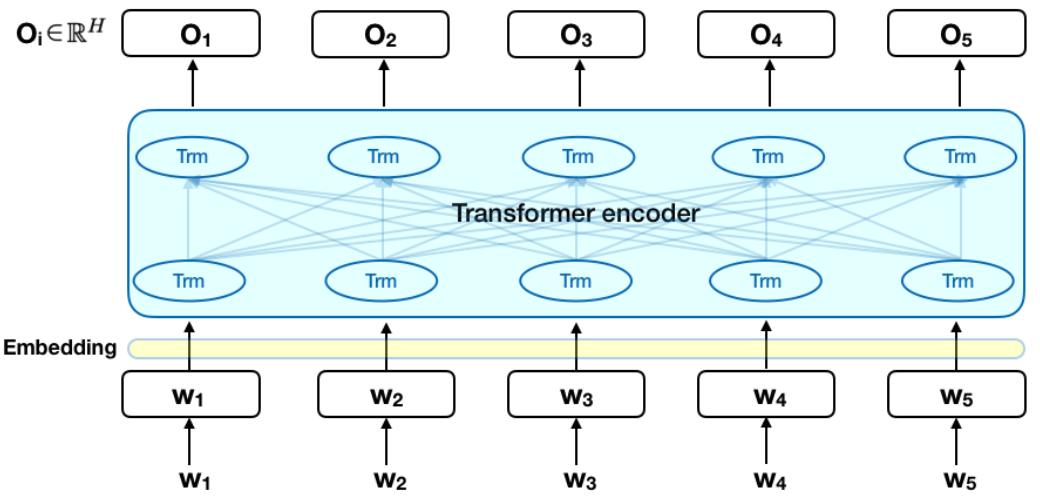w1, w2, w3, w4 and w4 are tokens (words in a sentence). These are converted into vectors which are taken from BERT vocab. Now it is passed through first layer of bert which gives 5 output vectors. Notice, that inside BERT layer, all words are connected with each other. This means that if we consider O1 output vector, it not only has a influence of W1, rather all the Ws has the influence on O1. All these influences are are calculated by weights of the layer. This helps the BERT to learn the context or helps it to learn about the words that are around it. Not only O1, but all the Os has the influence of all the Ws according to the weights. Now, if we do this 12 times, then BERTBASE of formed and if we do this 24 times BERTLARGE is formed.

If we dig a little deeper, the diagram below shows what is inside the "transformer encoder" layer shown above. It consists of Multi-head-attention module and feed forward attention module. The job of first module is to look at the words around that particular word and the job of second module is to take the output from the first module and pass that to a neural network and give us the encoded vector representation of the word. Output of the neural network is what Os are at the diagram above.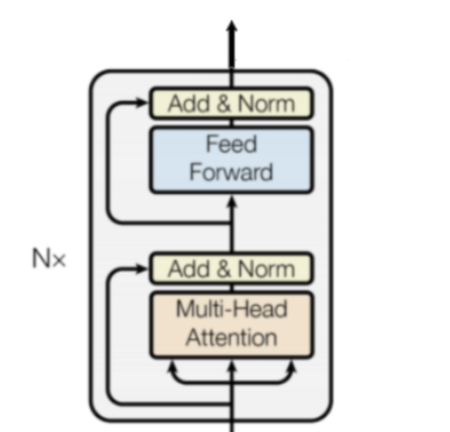This might have gotten a little intimidating, but I'll be adding some resources which will definitely help you to get a deeper insight into it. DO check them out!

Now, after getting enhanced or modified vectors after passing the input vectors to successive encoder layers, our job is to perform classification i.e., which class our input text belongs to. In BERT paper (referenced below), they gave a list of guidelines to follow when one want to use BERT for classification task. They said to add a classifier at the end of BERT output which was obvious. But they also said that the classifier we add at the last layer should only take the first vector (not the array of vectors that we get at the output for every word) which looked a little strange to me at first. There was no reason given whatsoever. What I think a plausible reason might be is that the way BERT works, there is no reason to use all the output vectors. This is because at every layer, BERT combines all the information from all the words to every single word. And it is happening at every layer. So after passing list of vectors to every layer, all the vectors have all the information of whole sentence. So, why use all of them, they are basically storing the same information. So, just use the first vector of the last layer. This is what I think why they advised us to do this. This seems logical but I might be wrong!

The diagram below depicts the classification task. Notice that our classifier ("fully connected") is just taking the first vector as an input (Not the whole array of vectors).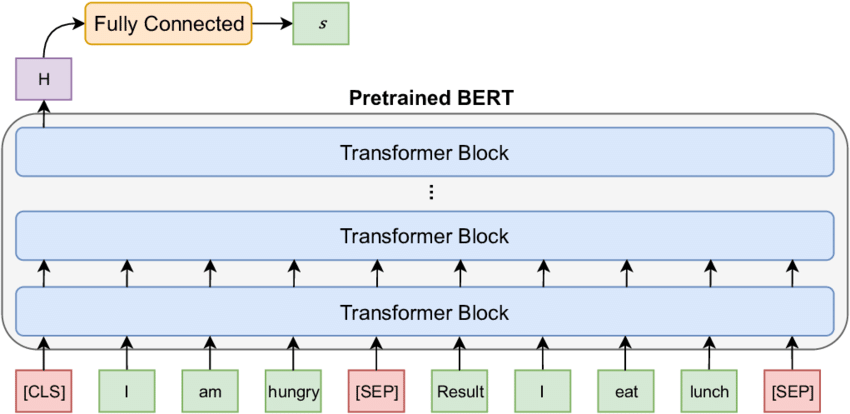Implementation

First I used some ML models like Support Vector Machines, Random Forest, etc for this classification task. Although, training was less time consuming but results were bad. Accuracy hovered around 30-40%. Then I explored some different Deep Learning based language models and settled on BERT.

To implement this model, we have used ktrain which is a lightweight wrapper for the deep learning library TensorFlow Keras to help build, train, and deploy neural networks and other machine learning models.

But, before that we need a dataset. Most commonly used dataset is Toefl11. The problem is that it is not available for public use and we need a license to use it. So, I found another dataset called 'italki' corpus which has 11 classes and each of them has around 1000 examples. Go to this link to download this dataset.

First task was to pre-process the data. There was many HTML tags, punctuations and numbers, unnecessary stop words and multiple spaces which needed to be taken care of. Calling the function shown below removes all these unnecessary items from the text.

df = pd.DataFrame(columns = ['text','type'])
import re
def preprocess_text(sen):
# Removing html tags
sentence = remove_tags(sen)
# Remove punctuations and numbers
sentence = re.sub('[^a-zA-Z]', ' ', sentence)
# Single character removal
sentence = re.sub(r"\s+[a-zA-Z]\s+", ' ', sentence)
# Removing multiple spaces
sentence = re.sub(r'\s+', ' ', sentence)
return sentence

TAG_RE = re.compile(r'<[^>]+>')

def remove_tags(text):
return TAG_RE.sub('', text)


We first store all the data (from data-frame) in the array and their corresponding labels. We do that by calling the pre-process function of the text, and then add it to the list with it's corresponding class.

reviews = []
y=[]
tags=['arabic','chinese','french','german','hindi','italian','japanese',
'korean','russian','spanish','turkish']
for index, row in data.iterrows():
filename='drive/My Drive/nlp/tokenized/'+row['Loc']
#str1 = ''.join(temp)
string=''
for i in temp:
string+=str(i)
pre = preprocess_text(string)
if(pre==' '):
continue
reviews.append(pre)
i=0
while i<len(tags):
if(tags[i]==row['Type']):
y.append(i)
break
i+=1
y=np.array(y)


Now, we convert our stored data in the array to training and test set. Image shown below is the snippet of the code which does that. 'test_size' has been set to 0.1 which divides the data into two parts: 90% to train set and 10% to text set.

from sklearn.model_selection import train_test_split
x_train, x_test, y_train, y_test = train_test_split(reviews, y, test_size = 0.1, random_state = 0)


Now, it's time to convert the input data (training and testing data) in the format used by BERT. We can simply add 'preprocess_mode='bert'' which can convert in the suitable format used by BERT.

Now, we are all set. Create a BERT model just by calling a function, assign number of batches you want to run and start training! (The image shown below is only for 4 classes). The image shows that it'll run for 4 epochs with learning rate set to 2e-5.

(x_train,  y_train), (x_test, y_test), preproc = text.texts_from_array(x_train=x_train, y_train=y_train,
x_test=x_test, y_test=y_test,
class_names=['arabic','russian','spanish','german'],
preprocess_mode='bert',
maxlen=350,
max_features=35000)


As we can see, our loss kept on decreasing and accuracy increased on every epoch, which shows training is going good. (The image shown below is only for 5 classes. Reason discussed below)

learner.fit_onecycle(2e-5, 4)


Now comes thee bad news. During training the model for 11 classes on google colab, it was taking 7-8 hours for each epoch! I even tried that but couldn't finish it for even 2 epoches. This is because colab generally disconnects the connection when it's GPU is being used for a long time. I tried it several times but couldn't do it.(Fun Fact : 4 GPUs can train BERT base for about 34 days!)

So, at last I trimmed the number of classes to 3. It took 10 minutes for each epoch. Then I increased the number of classes to 4 which took 13-14 minutes for each epoch which was modest as well. I then again tried to go further and increased the number of classes to 5 which was 15-16 minutes per epoch. I stopped here and all the results for every set is shown below.

• Results for 3 classes: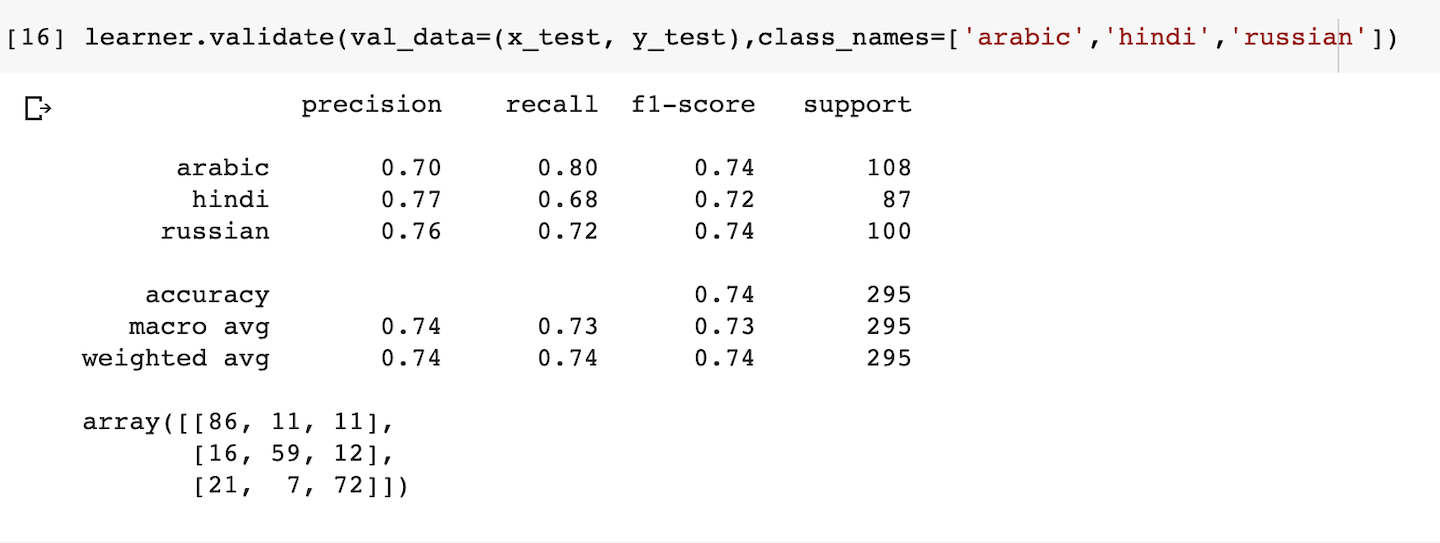• Results for 4 classes: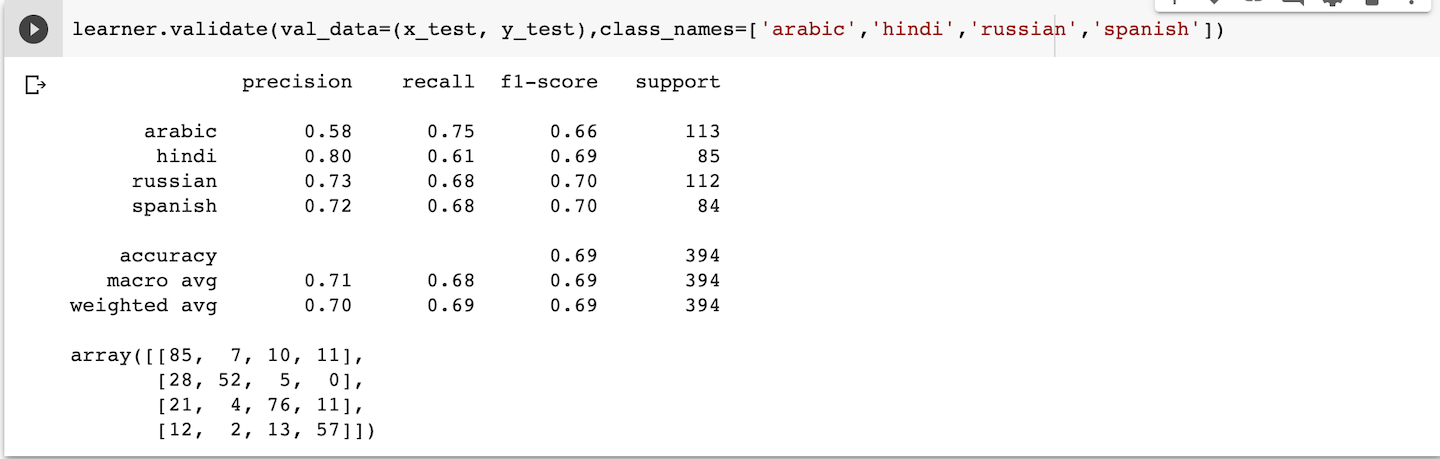• Results for 5 classes: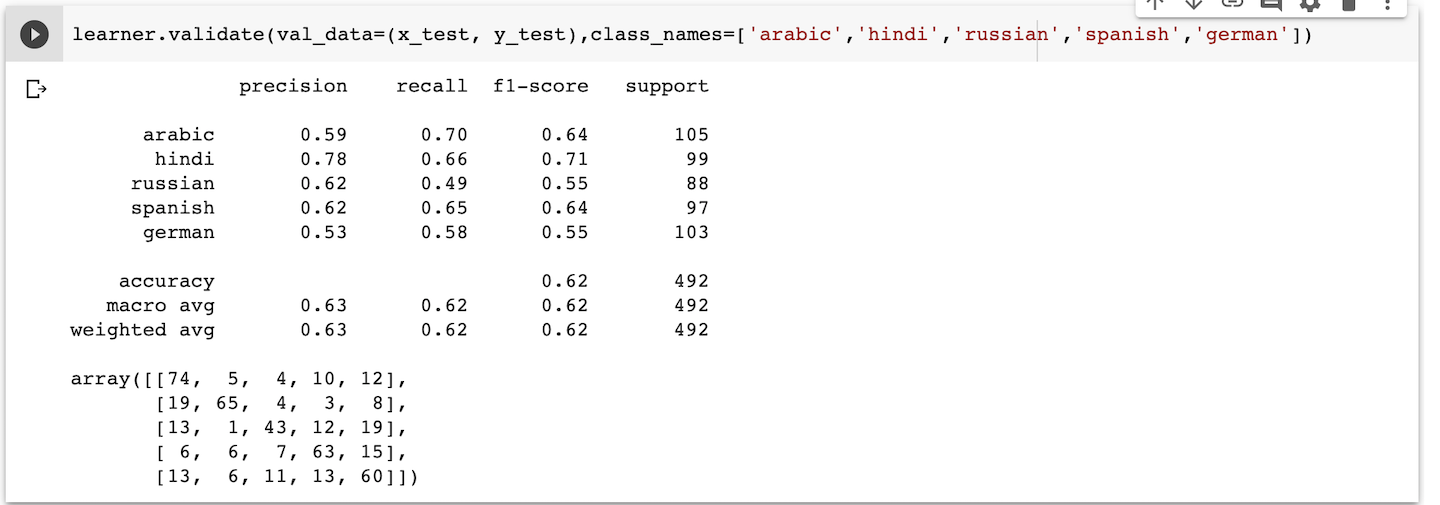Observations

It shows that accuracy falls from 74% for 3 classes to 69% for 4 classes to 62% for 5 classes. This is because we are only training our model to 4 epoch which was taking around 1-1.5 hours. When I pushed the number of epoches, google colab breaks the connection and time out error occurs.

On every results, we can see a 2d matrix which is called a confusion matrix. It shows how many texts were correctly classified and how many of them were misclassified. The diagonal elements shows the texts correctly classified and all other elements in the matrix shows number of texts that were misclassified.

So why is the accuracy a little low?

Well, NLI is not easy. It is not sentiment analysis where we have specific words like love, liked, enjoyed,good OR hate, worse, bad, stupid which are easy to classify. But for NLI, we have to look for hidden patters in the text which are common for people of one country which helps in showing the native language of the author.

And, to do that, we need large corpus (like Toefl11) and large training times for the model which is not possible with google colab. I was able to run the model for 4 epochs on colab. So, if you have the access to Toefl11 dataset and have a discrete GPU, do try to train it and compare the accuracy with what I achieved. It should easily surpass it.

Second, if you look at the second row of confusion matrix, that is for hindi. Most number of misclassified text were of Indians. What I think is due to different languages we speak all over India. NLI is basically the affect of your native language on your english. So, in India we all very diverse native languages; ranging from Tamil, Telgu, Urdu, Hindi, etc. So, a speaker of tamil language would have different affect on his english as compared to someone who speaks Urdu. That is why it is difficult to identify hidden similarity in English on Indians as they have different native languages and that's why our model performs a little worse on Hindi.

What you can do further in this?

As I said, you can do a lot if you have GPU. You can train your models longer. You could also use a custom model and train it from scratch if you have the resources to train. But, be aware, to train such a model from scratch, you need to have large (in-fact huge) training data. But good thing is, to train language models, we don't need labeled data. We use unlabeled text data (which is widely available across the Internet!)

REFERENCES